SSC  >  SSC CPO Mock Test (Quant Apt) 1

# SSC CPO Mock Test (Quant Apt) 1

Test Description

## 50 Questions MCQ Test SSC CPO & Constable - Mock Tests & Previous Year Papers | SSC CPO Mock Test (Quant Apt) 1

SSC CPO Mock Test (Quant Apt) 1 for SSC 2023 is part of SSC CPO & Constable - Mock Tests & Previous Year Papers preparation. The SSC CPO Mock Test (Quant Apt) 1 questions and answers have been prepared according to the SSC exam syllabus.The SSC CPO Mock Test (Quant Apt) 1 MCQs are made for SSC 2023 Exam. Find important definitions, questions, notes, meanings, examples, exercises, MCQs and online tests for SSC CPO Mock Test (Quant Apt) 1 below.
Solutions of SSC CPO Mock Test (Quant Apt) 1 questions in English are available as part of our SSC CPO & Constable - Mock Tests & Previous Year Papers for SSC & SSC CPO Mock Test (Quant Apt) 1 solutions in Hindi for SSC CPO & Constable - Mock Tests & Previous Year Papers course. Download more important topics, notes, lectures and mock test series for SSC Exam by signing up for free. Attempt SSC CPO Mock Test (Quant Apt) 1 | 50 questions in 30 minutes | Mock test for SSC preparation | Free important questions MCQ to study SSC CPO & Constable - Mock Tests & Previous Year Papers for SSC Exam | Download free PDF with solutions
 1 Crore+ students have signed up on EduRev. Have you?
SSC CPO Mock Test (Quant Apt) 1 - Question 1

### Ayesha's father was 38 years of age when she was born while her mother was 36 years old when her brother four years younger to her was born. What is the difference between the ages of her parents?

Detailed Solution for SSC CPO Mock Test (Quant Apt) 1 - Question 1 Mother's age when Ayesha's brother was born = 36 years.
Father's age when Ayesha's brother was born = (38 + 4) years = 42 years.
∴ Required difference = (42 - 36) years = 6 years.
SSC CPO Mock Test (Quant Apt) 1 - Question 2

### Find x and y x2 - xy = 4 and y2 - xy = -3

Detailed Solution for SSC CPO Mock Test (Quant Apt) 1 - Question 2

x2 - xy = y .....(1)
y2 - xy = -3 .....(2)
Subtracting equation (2) from equation (1) we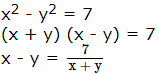Putting the value of x - y in equation (1) we get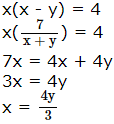Putting value of x in equation (2) we get,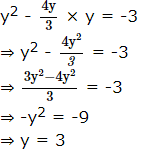putting value of y in equation (2) we get
y2 - xy = -3
⇒ 9 - 3x = -3
⇒-3x - -3 -9 = - 12
⇒ x = 4
So, (x, y) = (4, 3) or (-4, -3)

SSC CPO Mock Test (Quant Apt) 1 - Question 3

### An expression, which when divided by(x+1) gives (x2-x+1) as quotient and 3 as remainder, is equal to

SSC CPO Mock Test (Quant Apt) 1 - Question 4
Of the following quardratic equation, which is the one whose roots are 2 and -15?
Detailed Solution for SSC CPO Mock Test (Quant Apt) 1 - Question 4 Sum of roots = - 13
Product of roots = - 30
∴ Equation = x2 - x (sum of roots) + product of roots = 0
x2 + 13x - 30 = 0
SSC CPO Mock Test (Quant Apt) 1 - Question 5

Factorise a²-b²:

SSC CPO Mock Test (Quant Apt) 1 - Question 6

A square is inscribed in a circle of radius 10 units. The area of the circle not included in the square......(use π = 3.14)

Detailed Solution for SSC CPO Mock Test (Quant Apt) 1 - Question 6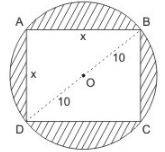Let the side of square be x unit.
sider the right triangle ABD
have x2 + x2 = (10 + 10)2
⇒ 2x2 = 400
x2 = 200
Area of square = 200 sq units
One of circle = πr2 = 22/7 x (10)2 = 314 sq units
One of shaded region not included in square
= 314 sq unit - 200 sq unit
= 114 sq unit.

SSC CPO Mock Test (Quant Apt) 1 - Question 7

Size of a tile is 9 inches by 9 inches. The number of tiles needed to cover a floor of 12 feet by 12 feet is

Detailed Solution for SSC CPO Mock Test (Quant Apt) 1 - Question 7

Length of floor = 12 feet
Breath of floor = 12 feet
So Area of floor = 12 x 12 = 144 feet
Area of tile = 9/12 x 9/12 = 9/16 feet
So the number of tile =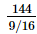= 256 tiles

SSC CPO Mock Test (Quant Apt) 1 - Question 8
An equilateral triangle has a side of 18 cm. Find the perimeter of triangle (in cm):
SSC CPO Mock Test (Quant Apt) 1 - Question 9

In a certain yr, the average monthly income of a person is Rs 3400 and that for the first 8 months is Rs 3160 and for the last 5 months is Rs 4120. The income in the eight month of the year is

Detailed Solution for SSC CPO Mock Test (Quant Apt) 1 - Question 9

x1 + x2 + ..... + x12 = 3400 x 12 .....(i)
x1 + ..... + x8 = 3160 x 8 .....(ii)
x8 + ..... + x12 = 4120 x 5 .....(iii)
from (i) and (ii)
x9 + ..... + x12 = 40800 - 25280 = 15520 .....(iv)
from (iii) and (iv) the income in the eight month of the year
x8 = 20600 - 15520 = Rs. 5080

SSC CPO Mock Test (Quant Apt) 1 - Question 10
Visitors to a show were charged Rs 15 each on the first day;Rs 7.50 on the second ;Rs 2.50 on the third and total attendence of three days were in the ratio 2:5:13 respectively. Find the average per person for the whole show.
SSC CPO Mock Test (Quant Apt) 1 - Question 11

if the pair of lines ax² + 2hxy + by² + 2gx + 2fy + c = 0

SSC CPO Mock Test (Quant Apt) 1 - Question 12
What will be the compound interest acquired on a sum of Rs 12000 for 3 years at the rate of 10% p.a?
SSC CPO Mock Test (Quant Apt) 1 - Question 13

A shopkeeper allows a discount of 10% on the marked price of an item but charges a sales tax of 8% on the discounted price. If the customer pays Rs 680.40 as the price including the sales tax, what is the marked price of the item?

Detailed Solution for SSC CPO Mock Test (Quant Apt) 1 - Question 13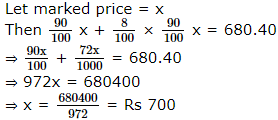SSC CPO Mock Test (Quant Apt) 1 - Question 14
The cube root of 1.061208 is
SSC CPO Mock Test (Quant Apt) 1 - Question 15
What is the difference between the niggest and the smallest fraction among 2/3, 3/4, 4/5 and 5/6?
SSC CPO Mock Test (Quant Apt) 1 - Question 16

Determine the value of ∠AOE​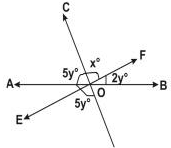SSC CPO Mock Test (Quant Apt) 1 - Question 17
A rectangular box is open on one side. The number of pairs of parallel planes of such a box is :
SSC CPO Mock Test (Quant Apt) 1 - Question 18

Two numbers,both greater than 29, have HCF 29 and LCM 4147. The sum of the numbers is

Detailed Solution for SSC CPO Mock Test (Quant Apt) 1 - Question 18

Product of numbers = 29 × 4147
Let the numbers be 29a and 29b
Then, 29a × 29b = (24 × 4147)
⇒ ab = 143
Now, co-primes with product 143 are (1, 143) and (11, 13)
So,the numbers are (29 × 1, 29 × 143) and (29 × 11, 29 × 13)
Since both numbers are greater than 29, the suitable pair is (29 × 11, 29 × 13)
i.e., (319, 377)
∴ Required sum = (319 + 377) = 696

SSC CPO Mock Test (Quant Apt) 1 - Question 19

AB and CD are two diameters of circles which are perpendicular to each other. The length of the chord BD is

Detailed Solution for SSC CPO Mock Test (Quant Apt) 1 - Question 19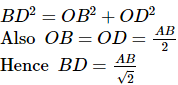SSC CPO Mock Test (Quant Apt) 1 - Question 20

A light house is 60 metres high with its base being at the sea level.If the angle of depression of a boat in the sea from the top of the light house is 15º,then the distance of the boat from the foot of the light house is equal to

SSC CPO Mock Test (Quant Apt) 1 - Question 21
The correct statement out of the following is

Detailed Solution for SSC CPO Mock Test (Quant Apt) 1 - Question 21 ∠A = ∠F; ∠B = ∠E and ∠C = ∠D
Hence, ∆ABC$\sim$∆FED.
SSC CPO Mock Test (Quant Apt) 1 - Question 22

How many digits in all are required to write numbers from 1 to 50?

Detailed Solution for SSC CPO Mock Test (Quant Apt) 1 - Question 22

1 to 9 = 9 (1 digit number)
10 to 50 = 41(2 digit number) = 41 x 2 = 82
total digits = 82 + 9 = 91

SSC CPO Mock Test (Quant Apt) 1 - Question 23

Suresh's monthly income is 30% more than that of Vinod. Vinod's monthly income is 20% less than that of Vinay.If the difference between the monthly incomes of Suresh and Vinay is Rs 800.What is the monthly income of Vinod?

Detailed Solution for SSC CPO Mock Test (Quant Apt) 1 - Question 23

Suresh = Vinod + 30% of Vinod
= 1.3 Vinod
Vinod = Vinay - 20% of Vinay
= 80% of Vinay
= 0.8 Vinay
∴ Suresh = 1.3 x 0.8 Vinay
= 1.04 Vinay
Now, Suresh - Vinay = 1.04 Vinay - Vinay
= 0.04 Vinay
= Rs 800 (given)
∴ Vinay = Rs 20000
∴ Vinod = 0.8 x 20000 = Rs 16000

SSC CPO Mock Test (Quant Apt) 1 - Question 24
Two-fifths of 30% of one-fourth of a number is 15. What is 20% of that number?
SSC CPO Mock Test (Quant Apt) 1 - Question 25
Two taps can fill a tank in 4 hours and 6 hours, respectively. A third tap can empty the tank in 3 hours. If all the three taps are opened how much time will it take to fill the tank?
SSC CPO Mock Test (Quant Apt) 1 - Question 26

A man sold two watches for Rs. 240 each on one he gained 20% and on the other he lost 20%. The gain or loss percent in the transaction is

Detailed Solution for SSC CPO Mock Test (Quant Apt) 1 - Question 26

S.P. of both the watches = Rs. 480
S.P. of each watch = Rs. 240
Gain = 20%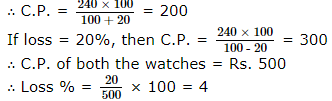SSC CPO Mock Test (Quant Apt) 1 - Question 27
If cost price is Rs 80,overhead is Rs 20 and selling price is Rs 120,then the profit percent is
SSC CPO Mock Test (Quant Apt) 1 - Question 28

Two sides of a triangle are 2√2cm and 2√3 cm and the angle opposite to the shorter side of the two is π/4. The largest possible length of the third side is

Detailed Solution for SSC CPO Mock Test (Quant Apt) 1 - Question 28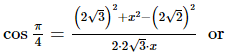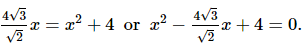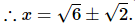SSC CPO Mock Test (Quant Apt) 1 - Question 29
One year ago the ratio of Karan's and Ankit's ages was 6:7, respectively. Four years hence this ratio would become 7:8. How old is Ankit?
SSC CPO Mock Test (Quant Apt) 1 - Question 30
If m is proportional to n and m=5 when n=4,then what is the value of m when n=18?
SSC CPO Mock Test (Quant Apt) 1 - Question 31

1³ - 2³ + 3³ - 4³ + ... + 9³ =

SSC CPO Mock Test (Quant Apt) 1 - Question 32
A sum of money doubles itself in 12 yrs if invested at simple interest. What is the rate of interest allowed on the investment?
SSC CPO Mock Test (Quant Apt) 1 - Question 33

(√72 − √18)÷√12 is equal to

SSC CPO Mock Test (Quant Apt) 1 - Question 34

The distance between two cities A and B is 330 km. A train starts from A at 8 a.m. and travels towards B at 60 km/hr. Another train starts from B at 9 a.m. and travels towards A at 75 km/hr. At what time do they meet?

Detailed Solution for SSC CPO Mock Test (Quant Apt) 1 - Question 34

The two trains meet x hrs after 8 a.m.
Distance covered by 1st train in x hrs + Distance covered by 2nd train in (x - 1) hrs = 330 km
60x + 75 (x - 1) = 330
60x + 75x - 75 = 330
x = 405/135 = 3
So, they will meet 3 hrs past 8 a.m. i.e., 11 a.m.

SSC CPO Mock Test (Quant Apt) 1 - Question 35
A alone can complete a work in 16 days and B alone in 12 days. Starting with A, they work on alternate days. The total work will be completed in :
SSC CPO Mock Test (Quant Apt) 1 - Question 36
If A+C=B, then tanA tanB tanC=
SSC CPO Mock Test (Quant Apt) 1 - Question 37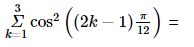SSC CPO Mock Test (Quant Apt) 1 - Question 38

The value of cos 12°+cos 84°+cos 156°+cos 132° is

SSC CPO Mock Test (Quant Apt) 1 - Question 39
A cubical vessel can hold 1331l of water. The length of side of the vessel in m is
SSC CPO Mock Test (Quant Apt) 1 - Question 40

A milk tanker cylindrical is shape having diameter 2 m and length 4.2 m supplies milk to two booths A and B in the ratio 3:2. Milk booth A is a rectangular vessel having base area as 3.96m2 and milk booth B is a cylindrical vessel having diameter 2m. The level of milk in vessels in booths A and B respectively are

SSC CPO Mock Test (Quant Apt) 1 - Question 41

Find the minimum number of cubes required to construct a cuboid of dimension 20cm × 16cm × 12cm ?

Detailed Solution for SSC CPO Mock Test (Quant Apt) 1 - Question 41

Volume of cuboid = 20 x 16 x 12 = 3840 cm3
Since minimum no. of cubes required
So length of cube = HCF of 20, 16, 12 cm = 4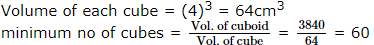SSC CPO Mock Test (Quant Apt) 1 - Question 42

The following line-graph gives the percent profit earned by two companies X and Y during the period 1996-2001. Study the line-graph and answer the questions that are based on it.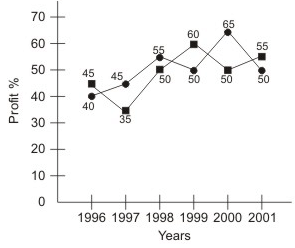Q. If the expenditure of Company Y in 1997 was Rs. 220 crores, what would its income in 1997 ?

Detailed Solution for SSC CPO Mock Test (Quant Apt) 1 - Question 42

Profit perecent of Company Y in 1997 = 35
Let the income of Company Y in 1997 be Rs x crores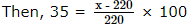⇒ x = 297
∴ Income of Company Y in 1997 = Rs 297 crores

SSC CPO Mock Test (Quant Apt) 1 - Question 43

The following line-graph gives the percent profit earned by two companies X and Y during the period 1996-2001. Study the line-graph and answer the questions that are based on it.Q. If the incomes of the two companies were equal in 1999, then what was the ratio of expenditure of Comapany X to that of Company Y in 1999 ?

Detailed Solution for SSC CPO Mock Test (Quant Apt) 1 - Question 43

Let the incomes of each of the two Companies X and Y in 1999 be Rs. x.
And let the expenditures of Companies X and Y in 1999 be E1 and E2 respectively.
Then, for Company X we have: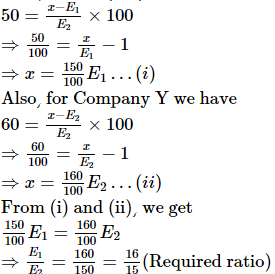SSC CPO Mock Test (Quant Apt) 1 - Question 44

The following line-graph gives the percent profit earned by two companies X and Y during the period 1996-2001. Study the line-graph and answer the questions that are based on it.Q. If the expenditure of companies X and Y in 1996 were equal and the total income of the two companies in 1996 was Rs. 342 crores, what was the total profit of the two companies together in 1996 ?

Detailed Solution for SSC CPO Mock Test (Quant Apt) 1 - Question 44

Let the expenditures of each companies X and Y in 1996 be Rs x crores
And let the income of Company X in 1996 be Rs z crores
So that the income of Company Y in 1996 = Rs (342 - z) crores
Then, for Company X we have: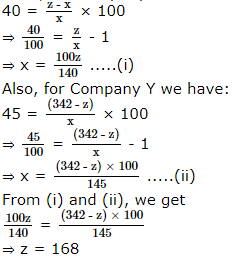Substituting z = 168 in (i), we get : x = 120
∴ Total expenditure of Companies X and Y is 1996 = 2x = Rs 240 crores
Total income of Companies X and Y in 1996 = Rs 342 crores
∴ Total profit = Rs (342 - 240) crores = Rs 120 crores

SSC CPO Mock Test (Quant Apt) 1 - Question 45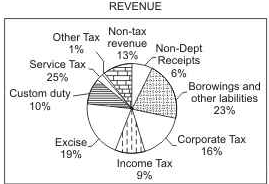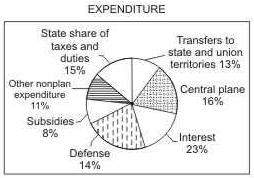The pie charts show estimated revenue and expenditure budgets for 2004-2005 for country X.
Notes: 1. Corporate tax revenues increased by Rs.2545 crore in the budget estimates for 2004-2005 over the estimates for 2003-2004.
2. Service tax brings in Rs.3990crore according to the budget for 2004-2005.
3. Total expenditure on defence increased by a whopping 28 percent from Rs.6030crore in 2003-2004 to Rs.7700crore in 2004-2005.

Q. The revenue generated by Custom duty, Income tax and Corporate tax according to the budget for 2004-2005 is.

SSC CPO Mock Test (Quant Apt) 1 - Question 46The pie charts show estimated revenue and expenditure budgets for 2004-2005 for country X.
Notes: 1. Corporate tax revenues increased by Rs.2545 crore in the budget estimates for 2004-2005 over the estimates for 2003-2004.
2. Service tax brings in Rs.3990crore according to the budget for 2004-2005.
3. Total expenditure on defence increased by a whopping 28 percent from Rs.6030crore in 2003-2004 to Rs.7700crore in 2004-2005.

Q. What is the percentage increase in the revenue generated by corporate tax is 2004-2005 over 2003-2004 according to the budgets?

SSC CPO Mock Test (Quant Apt) 1 - Question 47The pie charts show estimated revenue and expenditure budgets for 2004-2005 for country X.
Notes: 1. Corporate tax revenues increased by Rs.2545 crore in the budget estimates for 2004-2005 over the estimates for 2003-2004.
2. Service tax brings in Rs.3990crore according to the budget for 2004-2005.
3. Total expenditure on defence increased by a whopping 28 percent from Rs.6030crore in 2003-2004 to Rs.7700crore in 2004-2005.

Q. If the percentage distribution for the sectors from he Expenditure graph remain the same for the 2003-2004 and 2004-2005 budgets, then what is the difference in budgeted expenditures in 2003-2004 and 2004-2005?

SSC CPO Mock Test (Quant Apt) 1 - Question 48The pie charts show estimated revenue and expenditure budgets for 2004-2005 for country X.
Notes: 1. Corporate tax revenues increased by Rs.2545 crore in the budget estimates for 2004-2005 over the estimates for 2003-2004.
2. Service tax brings in Rs.3990crore according to the budget for 2004-2005.
3. Total expenditure on defence increased by a whopping 28 percent from Rs.6030crore in 2003-2004 to Rs.7700crore in 2004-2005.

Q. According to the budget for 2004-2005, the revenue generated by non-debt capital receipts is what percent of expenditure on subsides?

SSC CPO Mock Test (Quant Apt) 1 - Question 49

In the chart given below, A, B,C,D and E represents the costs of paper , printing , binding , miscellaneous and the royalty respectively in publishing a book.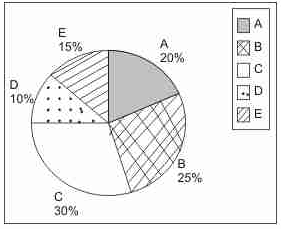Q. What is the angle of the pie chart showing the expenditure incurred on royalty ?

Detailed Solution for SSC CPO Mock Test (Quant Apt) 1 - Question 49

% of Royalty = 15%
∴ Angle made by Royalty = 360/100 × 15 = 54°

SSC CPO Mock Test (Quant Apt) 1 - Question 50

The following line-graph gives the percent profit earned by two companies X and Y during the period 1996-2001. Study the line-graph and answer the questions that are based on it.Q. The income of the companies X and Y in 2000 were in the ratio of 3 : 4 respectively. What was the respective ratio of their expenditures in 2000 ?

Detailed Solution for SSC CPO Mock Test (Quant Apt) 1 - Question 50

Let the incomes in 2000 of Companies X and Y be 3x and 4x respectively.
And let the expenditures in 2000 of Companies X and Y be E1 and E2 respectively.
Then, for Company X we have: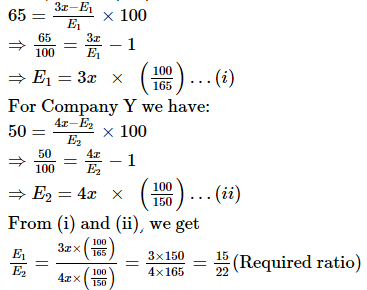## SSC CPO & Constable - Mock Tests & Previous Year Papers

1 docs|63 tests
 Use Code STAYHOME200 and get INR 200 additional OFF Use Coupon Code
Information about SSC CPO Mock Test (Quant Apt) 1 Page
In this test you can find the Exam questions for SSC CPO Mock Test (Quant Apt) 1 solved & explained in the simplest way possible. Besides giving Questions and answers for SSC CPO Mock Test (Quant Apt) 1, EduRev gives you an ample number of Online tests for practice

1 docs|63 tests